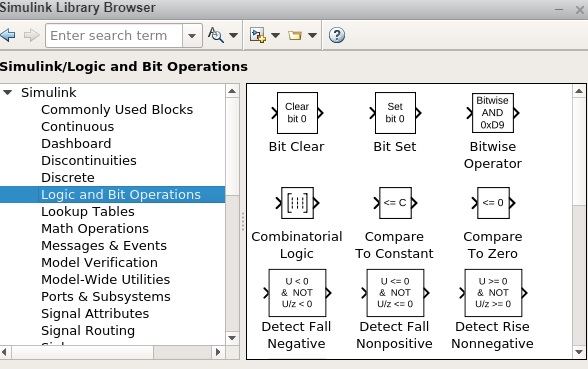Simulink is a simulation and model-based design environment for dynamic and embedded systems, which are integrated with MATLAB. Simulink was developed by a computer software company MathWorks.

It is a data flow graphical programming language tool for modelling, simulating and analysing multi-domain dynamic systems. It is basically a graphical block diagramming tool with a customisable set of block libraries.

Furthermore, it allows you to incorporate MATLAB algorithms into models as well as export the simulation results into MATLAB for further analysis.

• System-level design.

• Simulation.

• Automatic code generation.

• Testing and verification of embedded systems.

To get started with Simulink, type simulink in the command window as shown below −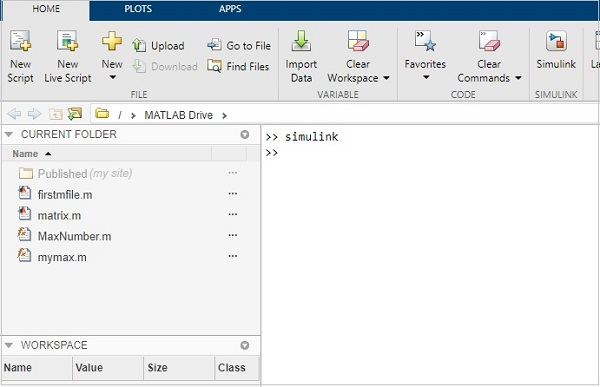It will open the Simulink page as shown below −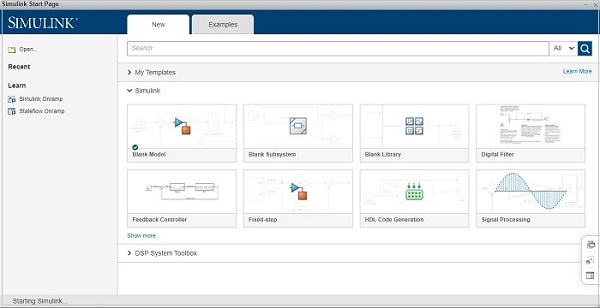You can also make use of Simulink icon present in MATLAB to get started with Simulink −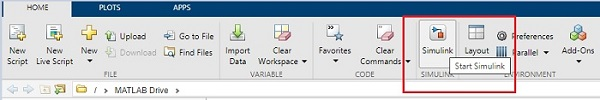When you start Simulink, you are navigated to the start page as shown below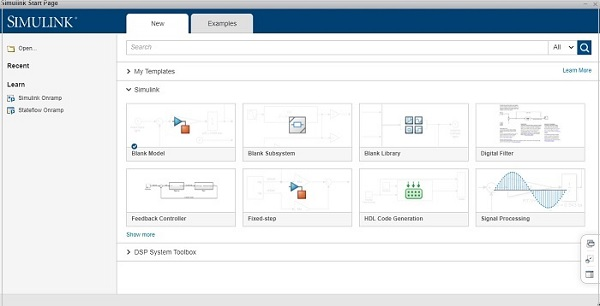Here you can create your own model, and also make use of the existing templates.

Click on the Blank Model and you will get a Simulink library browser that can be used to create your own model.

The screen for Blank model is as follows −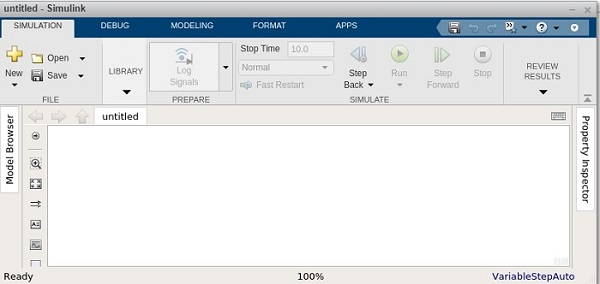Click on Library and it will display you the Simulink library as shown below −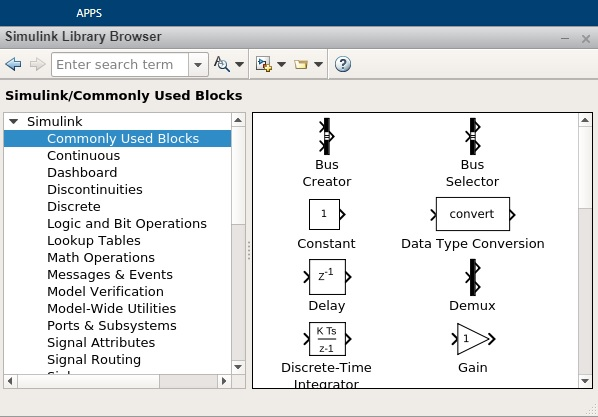The Simulink library browsers is a collection of many libraries. It offers Commonly Used Blocks, Continuous, Dashboard, Logic and Bit Operation, Math Operations etc.

Besides that, you will get other library list like Control system toolbox, DSP system toolbox etc.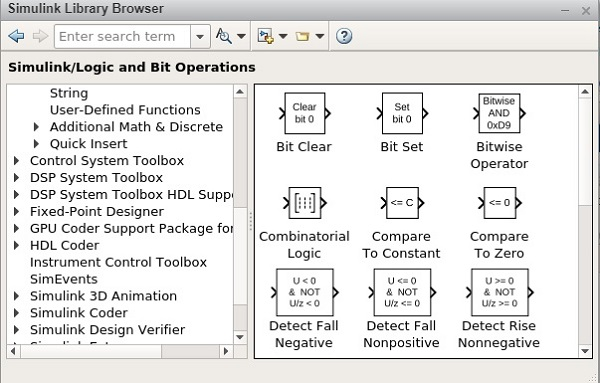Here is an example of Math operations library list −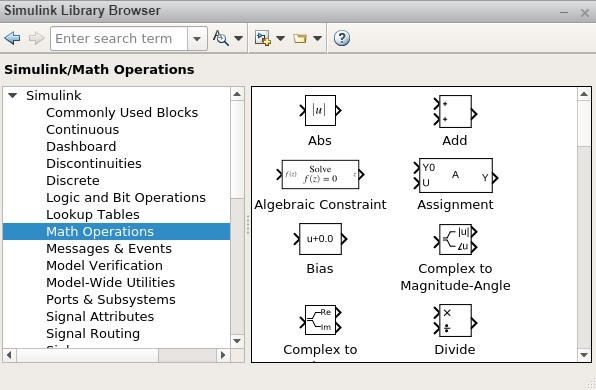It has Abs, Add, Algebraic Constraint, Assignment etc. that you can make use in your model.

Given below is an example of Logic and Bit Operations −